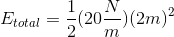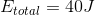# AP Physics C: Mechanics : Understanding Principles of Harmonic Motion

## Example Questions

### Example Question #1 : Understanding Principles Of Harmonic Motion

A 5.0 kg mass oscillates once. The total distance it travels is 1.5 m and it takes 4.0 s to travel that distance. What is its frequency of oscillation?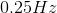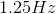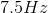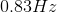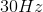Explanation:

Frequency is only based on the period of the oscillation; all the other given information is useless for this problem.  Using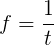, we can calculate that the frequency is 0.25 Hz.

### Example Question #1 : Understanding Principles Of Harmonic Motion

A mass oscillates on a spring with period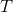. If the mass is doubled, what is the new period of oscillation?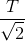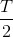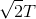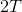Explanation:

The formula for the period of oscillation is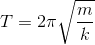.

When we double the mass, we get: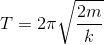Because the new factor of 2 is under the square root sign, and also in the numerator, the new period will be increased by.

### Example Question #21 : Motion

A mass is attached to the ceiling by a spring and hangs vertically downward. The mass is stretched downward so its amplitude isunits from its equilibrium position. What is the distance the mass travels during one full oscillation?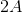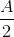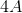Explanation:

When the mass is at point, it hasn't traveled at all. When it reaches the spring's equilibrium point, it has traveled a distance of. The mass then continues to a point that's equal to the initial distance traveled, but on the opposite side of the equilibrium point, so the total distance traveled so far is. The mass must then travel back to the starting point to complete the oscillation, so the total distance traveled is.

### Example Question #21 : Ap Physics C

A mass is attached to a spring, which is fixed to a wall. The mass is pulled away from the spring's equilibrium point and is then released. At what point does the mass experience its maximum kinetic energy?

Equal in all places

At the spring's equilibrium point

Where the mass started

Halfway through one full oscillation

The mass does not have kinetic energy

At the spring's equilibrium point

Explanation:

The formula for determining kinetic energy is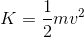So, kinetic energy will be greatest when the mass is moving most quickly. The force of the spring on the mass increases the mass's velocity until the spring’s equilibrium point, where the force of the spring acts against the motion of the mass, slowing it down. The mass is moving fastest at the spring's equilibrium point, so that's where its kinetic energy is greatest.

### Example Question #1 : Harmonic Motion

A mass is attached to a spring with a spring constant of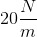.  The mass is moved 2 m from the spring's equilibrium point. What is the total energy of the system?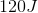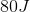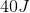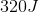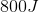Explanation:

The total energy of the system is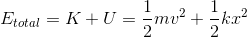but due to the face that the mass currently has no velocity, the kinetic energy term goes to zero.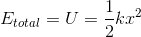Plugging in the given values, we can solve for the total energy of the system: